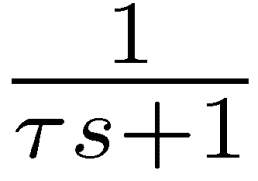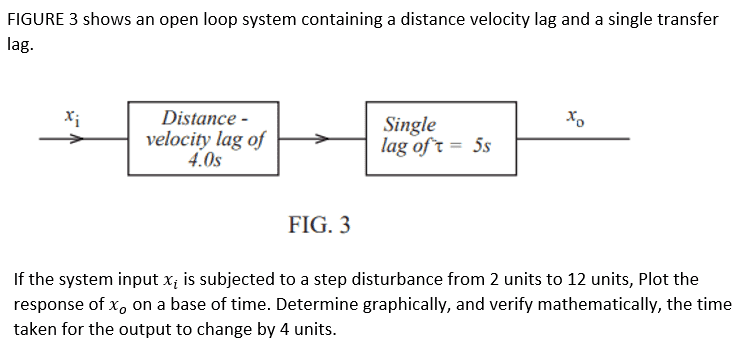# Open Loop system with distance-velocity lag and single transfer lag

• Engineering
Eng studies
Homework Statement:
Xi Xo
---->----[Distance - velocity lag of 4.0s]---->----[single transfer lag if t=5s]---->----

If the system input Xi is subjected to a step disturbance from 2 units to 12 units, plot the response of Xo on a base of time. Determine graphically, and verify mathematically, the time taken for the output to change by 4 units.
Relevant Equations:
Output change = input change (1-e ^-t/T)
Hi All, I have got as far as below, would anyone be able to go over this, I am a little confused with plotting this out onto a graph post equation

Any help would be appreciated, I am hoping to get better understanding before I move onto the next question

4 = 10 (1-e^-t/5)

t = -T in (output change/input change)

t = -5 In 6/10

t= 2.55 x 4s = 10.2

Homework Helper
Gold Member
Your question is very hard for me to interpret.
1) Is that "transfer lag" actually a pure delay of 5 seconds?
2) What is [Distance - velocity lag of 4.0s]?
I think it would help me understand if you show a signal diagram with the appropriate transfer blocks.

•DaveE
Eng studies
Your question is very hard for me to interpret.
1) Is that "transfer lag" actually a pure delay of 5 seconds?
2) What is [Distance - velocity lag of 4.0s]?
I think it would help me understand if you show a signal diagram with the appropriate transfer blocks.
Hi Thanks for your reply, Scratching my head with this a bit this is all the info given
Diagram is shown as below"Open loop system with a distance velocity lag and single transfer lag"

Question below

"If the system input Xi is subjected to a step disturbance from 2 units to 12 units, plot the response of Xo on a base of time. Determine graphically, and verify mathematically, the time taken for the output to change by 4 units."

Many Thanks

Homework Helper
Gold Member
That's the first step, but you need to do a lot more. It would be best if you made a more detailed diagram that answers the following questions:
1) Are distance and velocity two different inputs or is velocity derived from position (distance)? What exactly is ##x_i##?
2) What does "single transfer lag" mean? Is it a pure transport delay of 5 seconds or a lag?
You should use symbols for Laplace transformations like first-order lag:And pure transport delay:Eng studies
That's the first step, but you need to do a lot more. It would be best if you made a more detailed diagram that answers the following questions:
1) Are distance and velocity two different inputs or is velocity derived from position (distance)? What exactly is ##x_i##?
2) What does "single transfer lag" mean? Is it a pure transport delay of 5 seconds or a lag?
You should use symbols for Laplace transformations like first-order lag:
View attachment 323734
And pure transport delay:
View attachment 323735
I would like to able to answer your questions but what I have posted is all the information given on the question there is no more info provided
Will have a look into above and see how it goes

Homework Helper
Gold Member
Is the original question stated exactly as you posted in post #1? It's incomprehensible as it is. You should ask for clarification.
Another thing to ask is if it is (distance-velocity) lag of 4.0 sec. or distance - (velocity lag of 4.0 sec.)?

Eng studies
Hi Thanks for having a look at this, yes the question is as above, I thought id draw it our rather than copying and pasting direct from the homework statement but see belowStill not got any further with it

Homework Helper
Gold Member
Hi Thanks for having a look at this, yes the question is as above, I thought id draw it our rather than copying and pasting direct from the homework statement but see below

View attachment 323867

Still not got any further with it
Then there is a lot that is not sufficiently defined, including:
1) What is ##x_i##? Is it distance, velocity, or both?
2) Is the first block (Distance-velocity) lag of 4.0s? Or is it Distance - (velocity lag of 4.0s)?
3) The second block is called "single transfer lag of t=5s". It is very standard to talk about transport delays, where there is a pure delay of a certain amount, not a lag. Is that what it is? Or is it a Laplace transformation of a first-order lag?
IMO, it is not worth working on this without a clearer statement of the problem.

Eng studies
Then there is a lot that is not sufficiently defined, including:
1) What is ##x_i##? Is it distance, velocity, or both?
2) Is the first block (Distance-velocity) lag of 4.0s? Or is it Distance - (velocity lag of 4.0s)?
3) The second block is called "single transfer lag of t=5s". It is very standard to talk about transport delays, where there is a pure delay of a certain amount, not a lag. Is that what it is? Or is it a Laplace transformation of a first-order lag?
IMO, it is not worth working on this without a clearer statement of the problem.
No problem hence why I have seeked extra assistance on this, will take this back to clarify on the points made and hopefully get some answers to progress this